# Integral of arccos(x)

In this post I am going to show you how to integrate the inverse function of cos(x), acos(x), step-by-step. But first, let’s clear a few things.

What is arccos(x)?

Arccosine is the inverse function of cosine, therefore: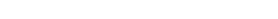These are equivalent forms: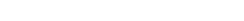The last form looks a lot like x^-1, which is equal to 1/x. In the case of trigonometric functions though, it just means inverse function, not one over that function. So,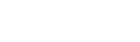but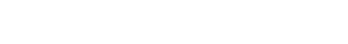Anyway, we are now ready to integrate!

Integral of arccos(x)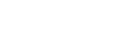The technique required for this integral is integration by parts:

Since we want to solve the integral of arccos(x), it would make no sense to let dv=arccos(x)dx. Also, arccos(x) is the same as 1*arccos(x), so we can integrate 1 and differentiate arccos(x), whose derivative is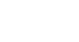.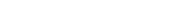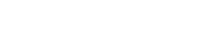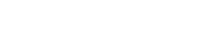Now we can use u-substitution, letting 1-x² be our u. When differentiating this, it will generate an x that cancels out with the one at the numerator; since we already used u in integration by parts, we will use t.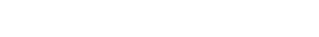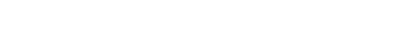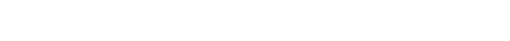Let’s now undo the substitution (remember that t=1-x²):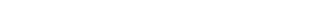Overall: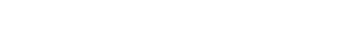And that’s it! Now try to solve this definite integral and leave the answer in the comments!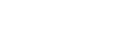I hope everything was clear and if you have any questions leave a comment and I’ll be happy to help!

Rating: 5 out of 5.

Join 31 other subscribers.